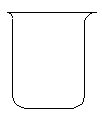# The Catalytic Decomposition of Hydrogen Peroxide, II

• Catalysis

## Demonstration

 The glass column contains: 3% hydrogen peroxide (H2O2) solution A mixture of dish soap and glycerine Food coloring. The other solution used is a saturated aqueous solution of KI. During the demonstration, the KI solution is added to the glass column.Observations

As soon as the catalyst is added, an eruption of foam flies out of the top of the gas column.

Explanation (including important chemical equations)

This demonstration is based on the decomposition of hydrogen peroxide into water and oxygen gas. Reactions like these that are both oxidations and reductions are known as disproportionation reactions:

2 H2O2(aq) -> 2 H2O(l) + O2(g)

Left on its own at room temperature, this reaction happens at a rate so slow that, for practical purposes, it may as well not even exist. A catalyst is added to speed things along. The KI added dissociates into K+ and I-, at which point the I- begins its work. The reaction pathway represented below has a lower activation energy than the straight decomposition represented above:

H2O2(aq) + I-(aq) -> OI-(aq) + H2O(l)

H2O2(aq) + OI-(aq) -> H2O(l) + O2(g) + I-(aq)

Note that the iodide ion is conserved in this reaction (it is not consumed in the sum of the reactions, and the same iodide ion could potentially go through many such cycles). This is the definition of a catalyst: a substance that increases the rate of a chemical reaction without being consumed in the reaction.